# 使用 TimeGAN 建模和生成时间序列数据

2021/09/09 09:14

## 时间序列数据 — 简要概述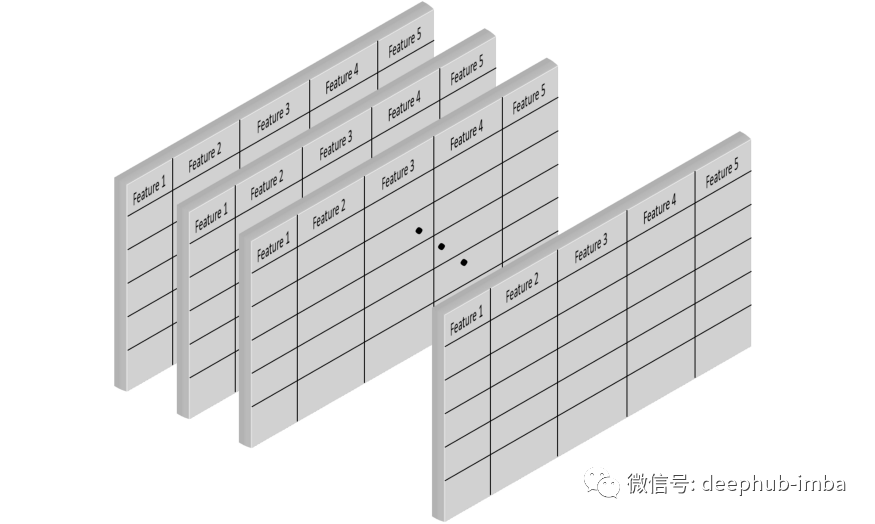## 能源数据集的案例研究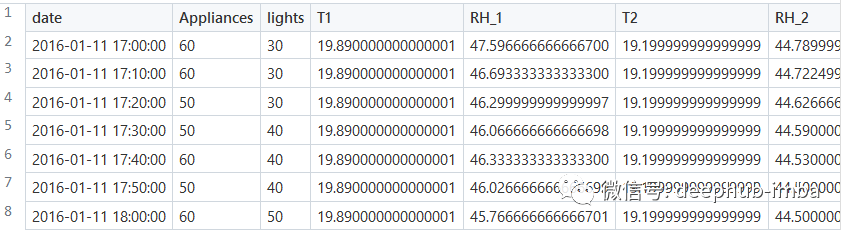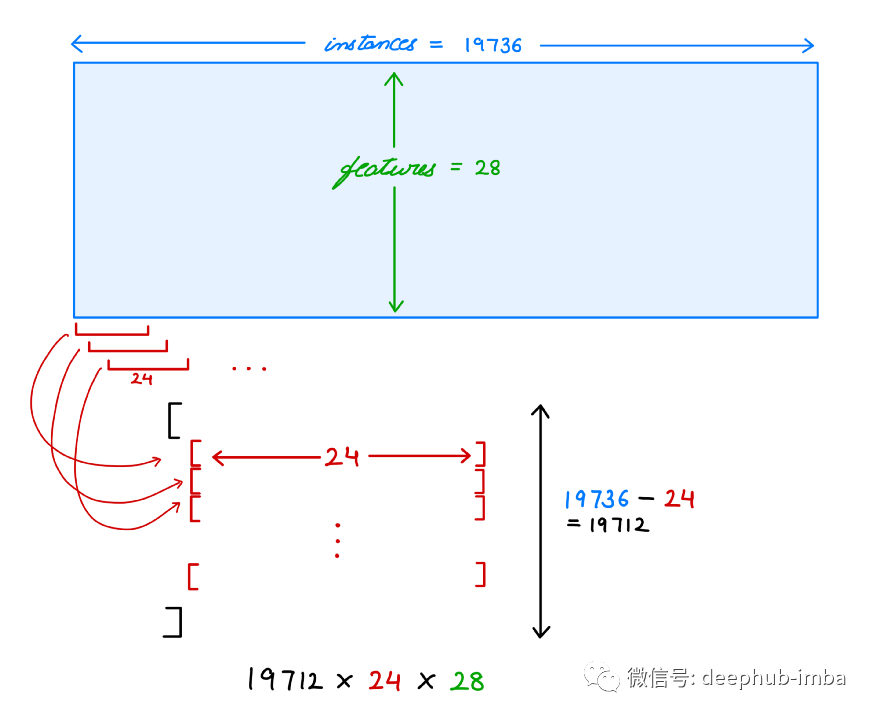## 使用TimeGAN生成时间序列数据

TimeGAN(时间序列生成对抗网络)是一种合成时间序列数据的实现。论文的作者也提供了相应的Python实现，在本文中，我们将使用0.3.0版本，这是撰写本文时的最新版本。

 pip install ydata-synthetic==0.3.0

 from ydata_synthetic.preprocessing.timeseries.utils import real_data_loading  file_path = "./data/energy_data.csv" energy_df = pd.read_csv(file_path)  try:     energy_df = energy_df.set_index('Date').sort_index() except:     energy_df=energy_df  # Data transformations to be applied prior to be used with the synthesizer model energy_data = real_data_loading(energy_df.values, seq_len=seq_len)  print(len(energy_data), energy_data.shape)

 from ydata_synthetic.synthesizers.timeseries import TimeGAN  synth = TimeGAN(model_parameters=gan_args, hidden_dim=hidden_dim, seq_len=seq_len, n_seq=n_seq, gamma=1) synth.train(energy_data, train_steps=500) synth.save('synth_energy.pkl')  synth_data = synth.sample(len(energy_data))

 seq_len = 24        # Timesteps n_seq = 28          # Features  hidden_dim = 24     # Hidden units for generator (GRU & LSTM).                     # Also decides output_units for generator  gamma = 1           # Used for discriminator loss  noise_dim = 32      # Used by generator as a starter dimension dim = 128           # UNUSED batch_size = 128  learning_rate = 5e-4 beta_1 = 0          # UNUSED beta_2 = 1          # UNUSED data_dim = 28       # UNUSED  # batch_size, lr, beta_1, beta_2, noise_dim, data_dim, layers_dim gan_args = [batch_size, learning_rate, beta_1, beta_2, noise_dim, data_dim, dim]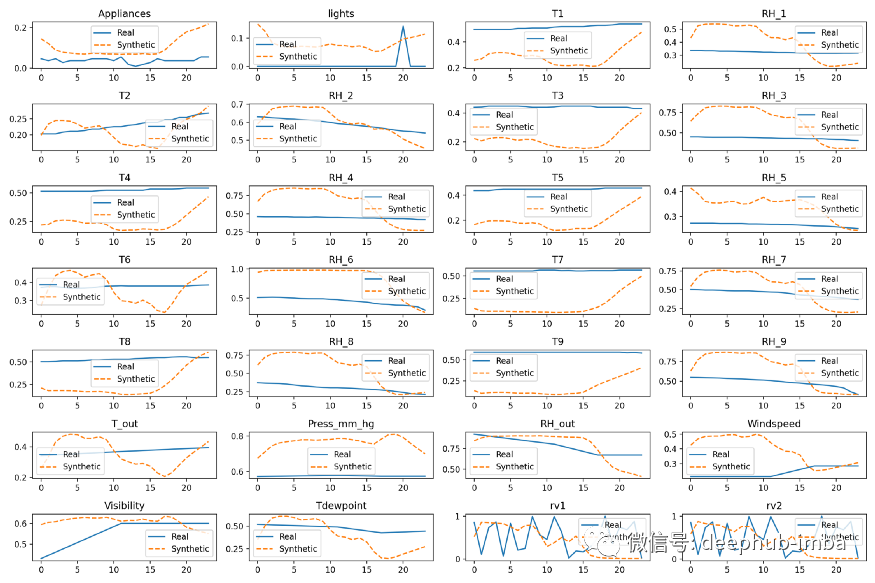## 评估和可视化

1. PCA——主成分分析

2. t-SNE — t-分布式随机邻居嵌入

 from sklearn.decomposition import PCA from sklearn.manifold import TSNE  sample_size = 250 idx = np.random.permutation(len(energy_data))[:sample_size]  # Convert list to array, but taking only 250 random samples # energy_data: (list(19711(ndarray(24, 28)))) -> real_sample: ndarray(250, 24, 28) real_sample = np.asarray(energy_data)[idx] synthetic_sample = np.asarray(synth_data)[idx]  # For the purpose of comparison we need the data to be 2-Dimensional. # For that reason we are going to use only two components for both the PCA and TSNE. # synth_data_reduced: {ndarray: (7000, 24)} # energy_data_reduced: {ndarray: (7000, 24)} synth_data_reduced = real_sample.reshape(-1, seq_len) energy_data_reduced = np.asarray(synthetic_sample).reshape(-1,seq_len)  n_components = 2 pca = PCA(n_components=n_components) tsne = TSNE(n_components=n_components, n_iter=300)  # The fit of the methods must be done only using the real sequential data pca.fit(energy_data_reduced)  # pca_real: {DataFrame: (7000, 2)} # pca_synth: {DataFrame: (7000, 2)} pca_real = pd.DataFrame(pca.transform(energy_data_reduced)) pca_synth = pd.DataFrame(pca.transform(synth_data_reduced))  # data_reduced: {ndarray: (14000, 24)} data_reduced = np.concatenate((energy_data_reduced, synth_data_reduced), axis=0)  # tsne_results: {DataFrame: (14000, 2)} tsne_results = pd.DataFrame(tsne.fit_transform(data_reduced))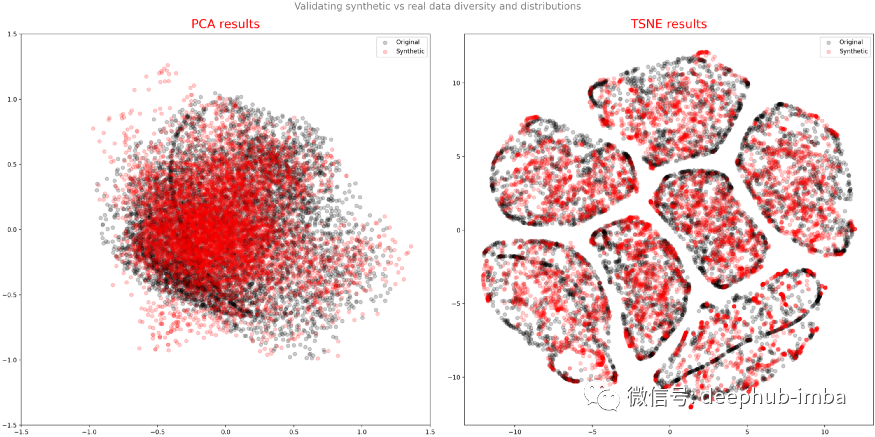## 总结点个 在看 你最好看！0 评论
0 收藏
0